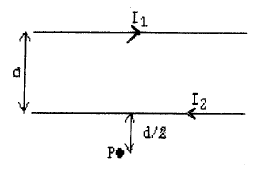# Problem: In the figure below, the two long straight wires are separated by a distance of d = 0.40 m. The currents are I1 = 1.0 A to the right in the upper wire and I2 = 8.0 A to the left in the lower wire. What is the magnitude and direction of the magnetic field at point P, that is distance d/2 = 0.20 m below the lower wire?Note: μ0 = 4π x 10-17 T x m/AA. B = 7.7 x 10-6 T, directed into the plane of the page.B. B = 8.3 x 10-6 T directed into the plane of the page.C. B = 7.7 x 10-6 T directed out of the plane of the page.D. B = 8.3 x 10-6 T directed out of the plane of the page.

🤓 Based on our data, we think this question is relevant for Professor Lumata's class at UTD.

###### Problem Details

In the figure below, the two long straight wires are separated by a distance of d = 0.40 m. The currents are I1 = 1.0 A to the right in the upper wire and I2 = 8.0 A to the left in the lower wire. What is the magnitude and direction of the magnetic field at point P, that is distance d/2 = 0.20 m below the lower wire?
Note: μ0 = 4π x 10-17 T x m/A

A. B = 7.7 x 10-6 T, directed into the plane of the page.

B. B = 8.3 x 10-6 T directed into the plane of the page.

C. B = 7.7 x 10-6 T directed out of the plane of the page.

D. B = 8.3 x 10-6 T directed out of the plane of the page.